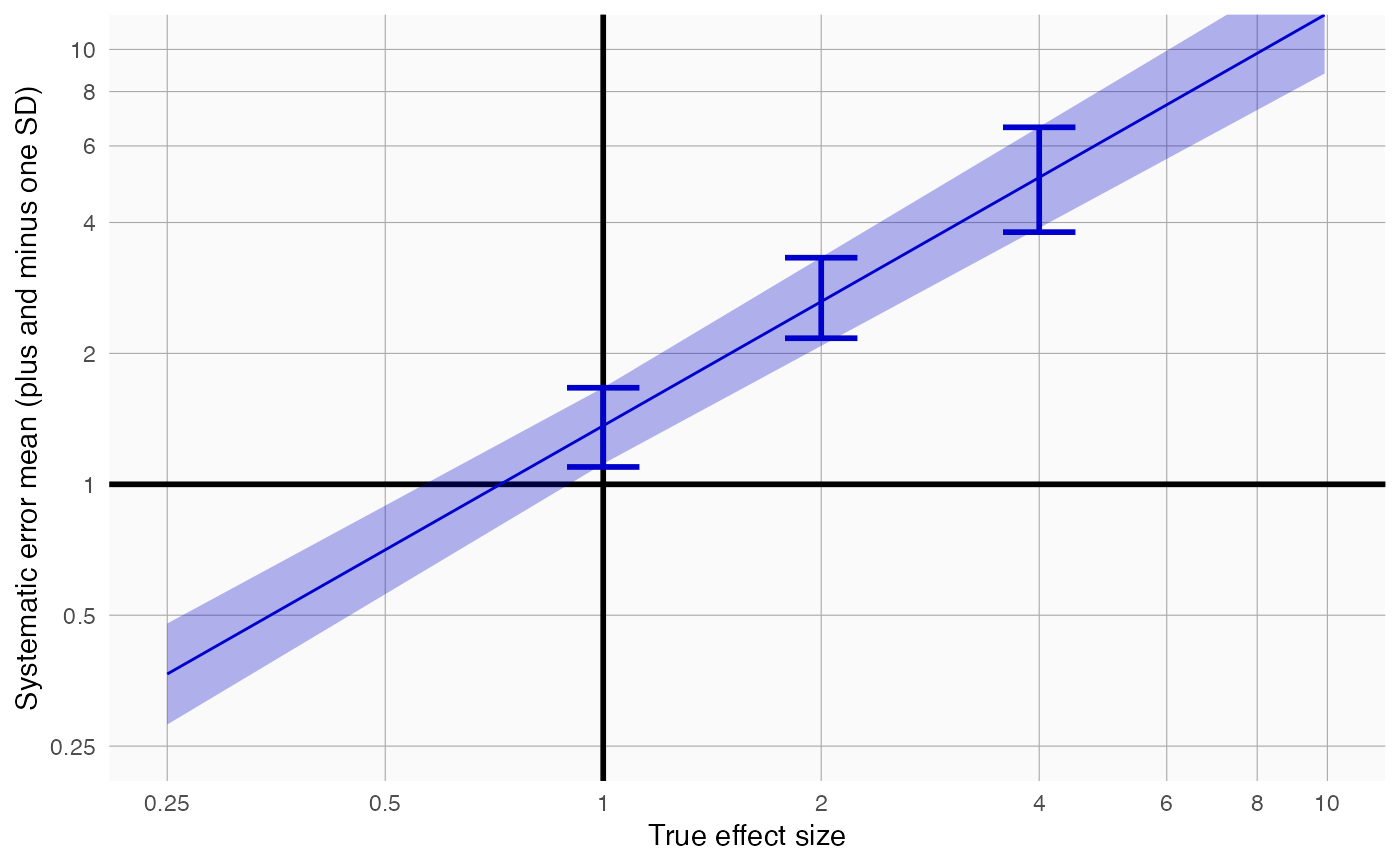plotErrorModel creates a plot showing the systematic error model.

plotErrorModel(
logRr,
seLogRr,
trueLogRr,
title,
legacy = FALSE,
fileName = NULL
)

## Arguments

logRr A numeric vector of effect estimates on the log scale. The standard error of the log of the effect estimates. Hint: often the standard error = (log() - log())/qnorm(0.025). The true log relative risk. Optional: the main title for the plot If true, a legacy error model will be fitted, meaning standard deviation is linear on the log scale. If false, standard deviation is assumed to be simply linear. Name of the file where the plot should be saved, for example 'plot.png'. See the function ggsave in the ggplot2 package for supported file formats.

## Value

A Ggplot object. Use the ggsave function to save to file.

## Details

Creates a plot with the true effect size on the x-axis, and the mean plus and minus the standard deviation shown on the y-axis. Also shown are simple error models fitted at each true relative risk in the input.

## Examples

data <- simulateControls(n = 50 * 3, mean = 0.25, sd = 0.25, trueLogRr = log(c(1, 2, 4)))
plotErrorModel(data$logRr, data$seLogRr, data\$trueLogRr)